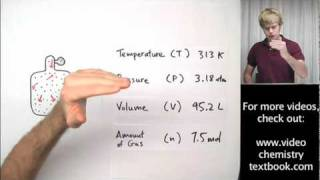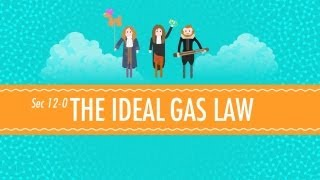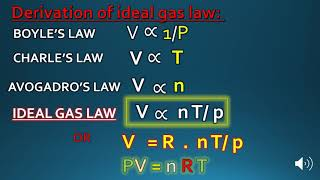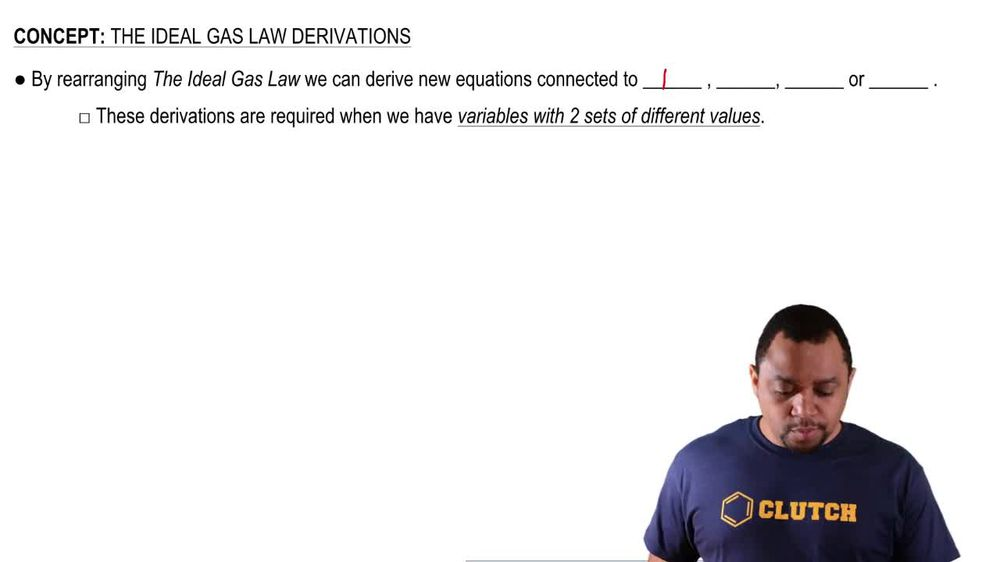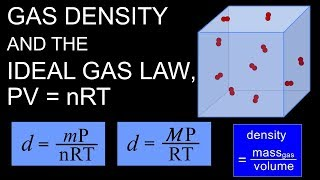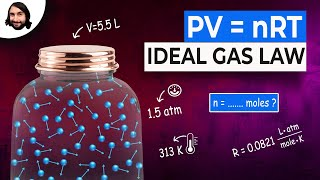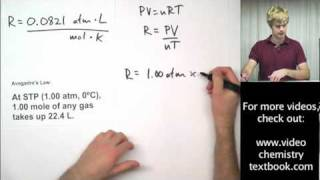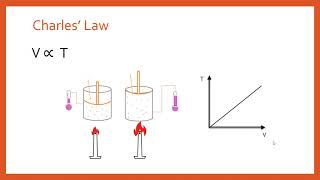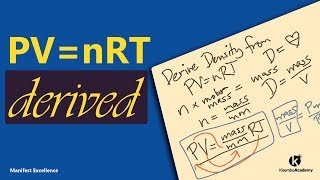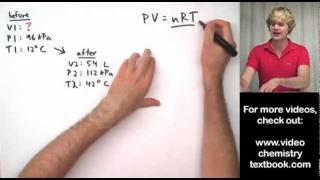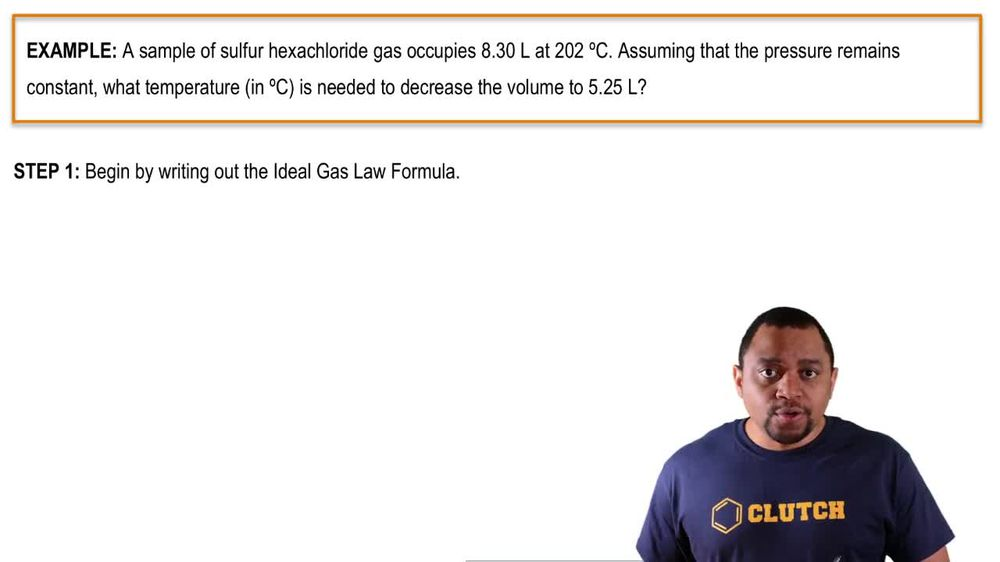Start typing, then use the up and down arrows to select an option from the list.
1. 7. Gases2. The Ideal Gas Law Derivations# The Ideal Gas Law Derivations

by Jules Bruno
147 views
1
0
By rearranging the ideal gas law, we can derive new equations connected to pressure volume, moles and temperature. We're gonna say these derivations are required when we have variables with two sets of different values. So basically we'll be we'll be dealing with the question where the ideal gas laws in play and within the question they may give you two pressures and to temperatures or two volumes with two moles. That's when we have to do one of these types of derivations. So just remember, we're still utilizing the ideal gas law, which is changing it a bit when we're dealing with two pressures or two volumes, two moles or to temperatures within any given question. Now that we've seen this, let's go on to our example question in the next video.